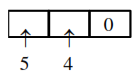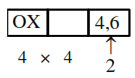# The number of three-digit even numbers, formed

Question:

The number of three-digit even numbers, formed by the digits $0,1,3,4,6,7$ if the repetition of digits is not allowed, is

Solution:

(i) When '0' is at unit placeNumber of numbers $=20$

(ii) When 4 or 6 are at unit placeNumber of numbers $=32$

So number of numbers $=52$